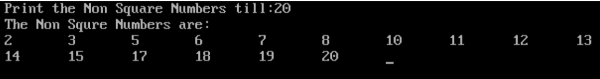# Print the Non Square Numbers in C

CServer Side ProgrammingProgramming

## Program Description

The square of a number is that number times itself.

A square number or perfect square is an integer that is the square of an integer;

The perfect squares are the squares of the whole numbers

1, 4, 9, 16, 25, 36, 49, 64, 81, 100

Here are the square roots of all the perfect squares from 1 to 100.

√1 = 1 since 12 = 1
√4 = 2 since 22 = 4
√9 = 3 since 32 = 9
√16 = 4 since 42 = 16
√25 = 5 since 52 = 25
√36 = 6 since 62 = 36
√49 = 7 since 72 = 49
√64 = 8 since 82 = 64
√81 = 9 since 92 = 81
√100 = 10 since 102 = 100

A non-perfect square is every number that is not the result of squaring an integer with itself.

The below numbers are non-perfect square numbers

2,3,5,6,7,8,10,11,12,13,14,15,17,18,19,20,21,22,23,24,26 etc…

## Algorithm

Check all numbers from 1 to the user specified number.
Check if it is perfect square or not.
If not a perfect square, print the Non Perfect Square Number.

## Example

/* Program to print non square numbers */
#include <stdio.h>
#include <math.h>
int main() {
int number,i,x;
int times = 0;
clrscr();
printf("Print the Non Square Numbers till:");
scanf("%d", &number);
printf("The Non Squre Numbers are:");
printf("\n");
for(i = 1;times<number;i++,times++){
x = sqrt(i);
if(i!=x*x){
printf("%d\t", i);
}
}
getch();
return 0;
}

## Output